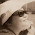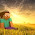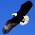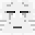## Wednesday, 27 February 2013

### Algebra... Help! What's Wrong?

Jane did the following:
• 3a + b = 3ab
• 2s + 4t = 6st
Do you think Jane is correct in her algebraic manipulations?
If yes, please write down examples to show that her answer is correct.

If not, explain to Jane her mistakes and help her to correct.

1.no, i do not think jane is correct in her algebraic expressions.

for the first equation, pretend that a stands for apple and b stands for grape. so 3a + b is 3 x apple + 1 grape, therefore writing it as 3ab is wrong as 3ab is 3 x apple x grape and you cant multiply apples and grapes because they are completely diff things

for the second equation, pretend that s stands for car and t stands for bus. so 2s + 4t is 2 cars and 4 busses, therefore writing it as 6st is wrong as that means 6 x cars x busses and you cant multiply two completely diff things.

[ sorry for silly explanation =.=" idk how to explain properly ]

1.Good analogy :)

2.No,Jane is wrong.

For the first question, the answer should be (3a+b).Jane wrote 3ab which shows that b is multiplied with 3a and not added to.So thus the answer should be (3a+b).

For the second question,the answer should be (2s+4t) because if the answer is 6st like what Jane wrote, it means that s and t are multiplied together and not added.

3.No.
3ab is not equal to 3a+b.3ab is 3xaxb.It should be 3a+b
2s+4t si not the same as 6st.6st is 6xsxt. It should be 2s+4t.

4.No.
For the first question,3a+b should give (3a)+b, 3ab is gotten when 3a*b.
For the second question,2s+4t should give (2s+4t).

5.No. Writing the answer as 3ab is wrong as its a multiplication but the question states addition.
For the second qn the answer is wrong as Jane should not associate two different things and add them together.

6.Both the answers are wrong.
1) She had to add instead of multiplying. Ans is: 3a+b (the answers are same as the question as they cannot be further simplified)
2) She added 2 and 4 and multiplied s and t. Ans is: 2s+4t

7.Jane is dumb. How come addition became multiplication??? We cannot just change the question from addition to multiplication. :P

8.She is writing her answers in multiplication when it should be addition. She should not just put the number right there but add a + sign and the answer will be correct.( Q1) for Q2, she should not add to unknowns together like that she should leave it as it is because the unknowns are very likely to have different values.

9.No i do not agree with Jane.
-3a+b does not equal to 3ab as 3ab expanded is 3*a*b while 3a+b equals to 3*a+b, which is different.
-2s+4t does not equal to 6st as 6st=2s TIMES 4t. She used multiplication instead of addition, which is not what the question is asking for.

10.2s + 4t = 6st

I do not agree with Jane.

3a + b = 3 a + b and not 3ab (3ab stands for 3 x a x b, which is not what the question was asking anymore)

For 2s + 4t, she added 2 + 4 and multiplied s and t, and the question did not ask for multiplication of anything.

11.Jane is wrong.

1. 3a + b = 3ab

3ab is referring to 3*a*b and not addition. Therefore the statement is absolutely wrong

2. 2s + 4t = 6st

Same as the equation above. 6st is referring to 6*s*t and not adding values of s and t together.

12.Jane is wrong

1. 3a + (1)b = (3a)+b, and not 3ab

2. 2s + 4t

In the question, its not specified if s and t has the same value hence jane cannot add both s and t together with that assumption.

13.I do not agree with jane.
1.3a + 1b =3a + b
2s + 4t = 2s + 4t

14.Jane is wrong as this rule is only applied during multiplication of algebra terms and not addition

15.NO 3ab=3xaxb
and 6st is 6xsxt